# What is the volume of your next DIY project?## Learn how to calculate volume of different shapes

### What is volume?

Volume is the amount of space that a three-dimensional object occupies. It is calculated by using this basic formula: width x length x height or depth. Now if you’ve just figured out how to calculate the area of a space, don’t worry we’ll help you figure out volume as well. Technically you’re already two thirds of the way there.

### Why do you need to know the volume?

Whether you are building a fence, placing sand underneath pavers, or preparing screed for your bathroom renovation, it’s important to know the right volume of the job to ensure the right amount of materials are used.

Product Packaging will often tell you how many cubic metres one bag will fill. So, knowing the volume will make it easier to figure out how many bags you’ll need for your job.

A tip – keep all the measurements the same – all in millimetres or centimetres or metres. This will make your calculations way easier! And a quick note on converting to metres: there is 1000mm in 1m and 100cm in 1m.

Now before we jump into the maths for calculating the volume of different shapes, here is an online volume calculator if maths is just not your thing.

### Measuring Volume for Rectangles and Squares

Let’s start with the simplest shapes – rectangles and squares. To calculate the volume you will need to know the width, length and height or depth of the space. If you are working with a square, all three numbers will be the same.

Volume of a Rectangle or Square = width × length × height/depth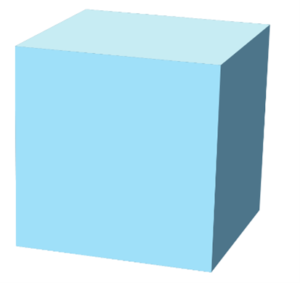Example: We are wanting to calculate the amount of concrete need to create a slab for the BBQ to sit on. The measurements are 70mm deep, 500mm wide by 800mm long.

Before we add in the figures, let’s quickly convert them to metres.

Depth = 70 ÷ 1000 = 0.07m

Width = 500 ÷ 1000 = 0.5m

Length = 800 ÷ 1000 = 0.8m

0.8 × 0.5 × 0.07

0.028m³

One 20kg Easy Mix Concrete bag makes 0.01m³ of concrete, so we divide 0.028 by 0.01 to find out how many bags we’ll need. The answer is 2.8 bags of concrete will make our BBQ foundation.

### Measuring volume for Prisms, Cylinders, Cones, Pyramids and Spheres

If simple shapes are not part of your design, we’ve got you covered. This next section will show you how to calculate the volume of more complex 3D shapes including prisms, cylinders, cones, pyramids and spheres. The good news is that these objects share formulas they are relatively simple.

We’ll start with the easiest formula first – the one for prisms and cylinders.

Volume of a Prism or Cylinder = Area of the Base × Height of the Object

Example: you are wanting to fill in some piping with concrete and need to know how many bags you will need. The diameter of the pipe is 250mm and it is collectively 1.5m long.

To calculate the volume of a cylinder, we need to use the following equation.

Volume of a cylinder = (πr²) × Height of the object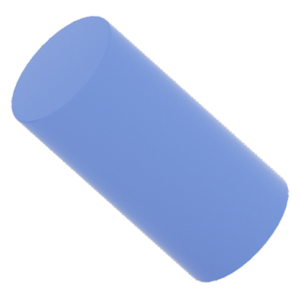Let’s convert our measurements to metres.

Diameter of the circle = 250 ÷ 1000 = 0.25m

Radius (r) of a circle = 0.25 ÷ 2 = 0.125m

(π × 0.125²) × 1.5

0.049087 × 1.5

0.0736m³

One 20kg Easy Mix Concrete bag makes 0.01m³ of concrete, so we divide 0.0736 by 0.01 to find out how many bags we’ll need. The answer is 7.36 bags of concrete will fill our length of pipe.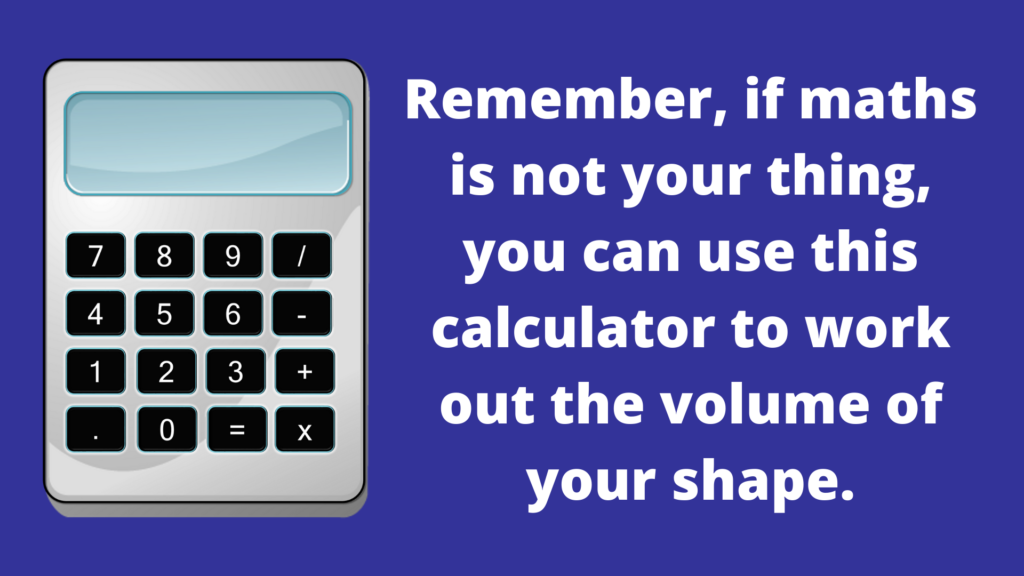Now let’s move onto the formula for Cones and Pyramids.

Cubic metres of a Cone or Pyramid = Area of the Base × Height of the Object × 1⁄3

Example: you want to make a feature piece for the garden out of concrete and you are tossing up between a cone or pyramid to incorporate into the design.

If you go ahead with the cone, the base needs to have a diameter of 50cm. While the pyramid would have all three sides at 25cm. Both shapes would have a height of 1m.

To calculate the volume of the pyramid, we’re looking at the following calculation.

Volume of a pyramid = (½bh) × Height of the object × 1⁄3Let’s convert our measurements to metres.

Sides of the pyramid base = 25 ÷ 100 = 0.25m

Height of the pyramid = 1m

(½ × 0.25 × 0.25) × 1 × 1⁄3

0.03125 × 1 × 1⁄3

0.0104m³

Now let’s do the cone; you’ll need to use this formula. The only difference between the two is how you calculate the area of the base.

Volume of a cone = (πr²) × Height of the object × 1⁄3Our measurements in metres.

Diameter of the circle = 50 ÷ 100 = 0.5m

Radius (r) of a circle = 0.5 ÷ 2 = 0.25m

Height of the cone = 1m

(π × 0.25²) × 1 × 1⁄3

0.0625 × 1 × 1⁄3

0.0208m³

One 20kg Easy Mix Concrete bag makes 0.01m³ of concrete. The pyramid requires 0.0104m³ of concrete and the cone needs 0.0208m³ so we divide those numbers by 0.01 to find out how many bags we’ll need. The answer is 1.04 bags of concrete will fill the pyramid and 2.08 bags will fill the cone.Okay, so now that you have cones and pyramids down pat, let’s look at spheres.

The equation you will need to calculate the volume of a sphere is:

Volume of a sphere = 4⁄3 × π × r³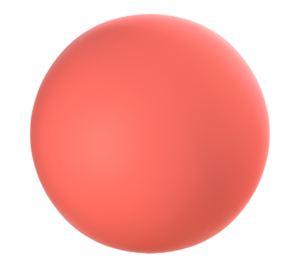Example:  you are wanting to make spheres to be included as part of your water feature in the back yard. The spheres need to have a diameter of 60cm for them to fit into the design.

Before we begin calculating, remember to convert your measurement to metres.

Diameter of the sphere = 60 ÷ 100 = 0.6m

Radius (r) of a circle = 0.6 ÷ 2 = 0.3m

4⁄3 × π × 0.3³

0.11309m³

One 20kg Easy Mix Concrete bag makes 0.01m³ of concrete, so we divide 0.11309 by 0.01 to find out how many bags we’ll need. The answer is 11.309 bags of concrete to create one sphere for the water feature.

Every Easy Mix bag will tell you how many cubic metres it will create/or fill to help you with your calculations.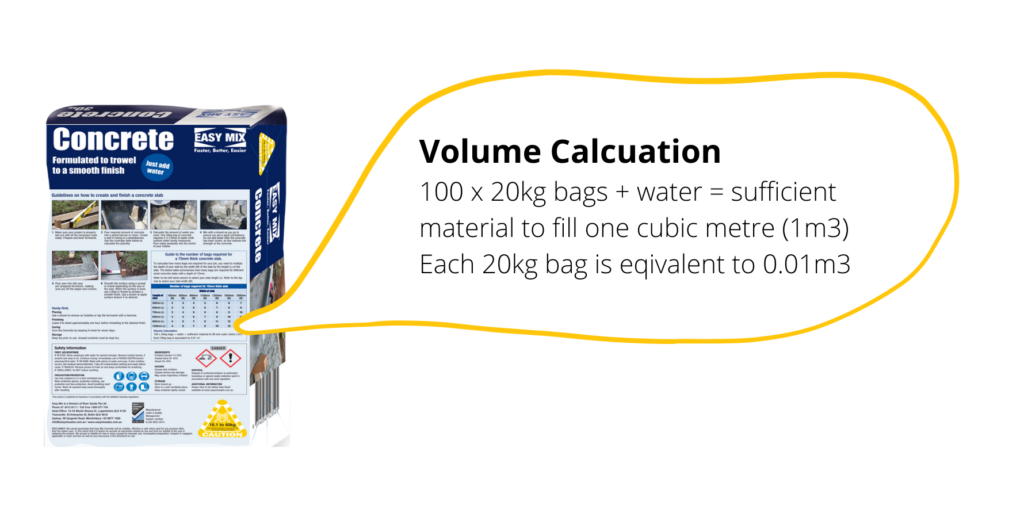Remember, if maths is not your thing, you can use this calculator to work out the volume of your shape.

Check out our blog for other DIY tips and guides and project inspiration.

## Laying and Filling Pavers

Laying and filling pavers is easy with the Easy Mix range of landscaping sand and gravel products. Before You Start…